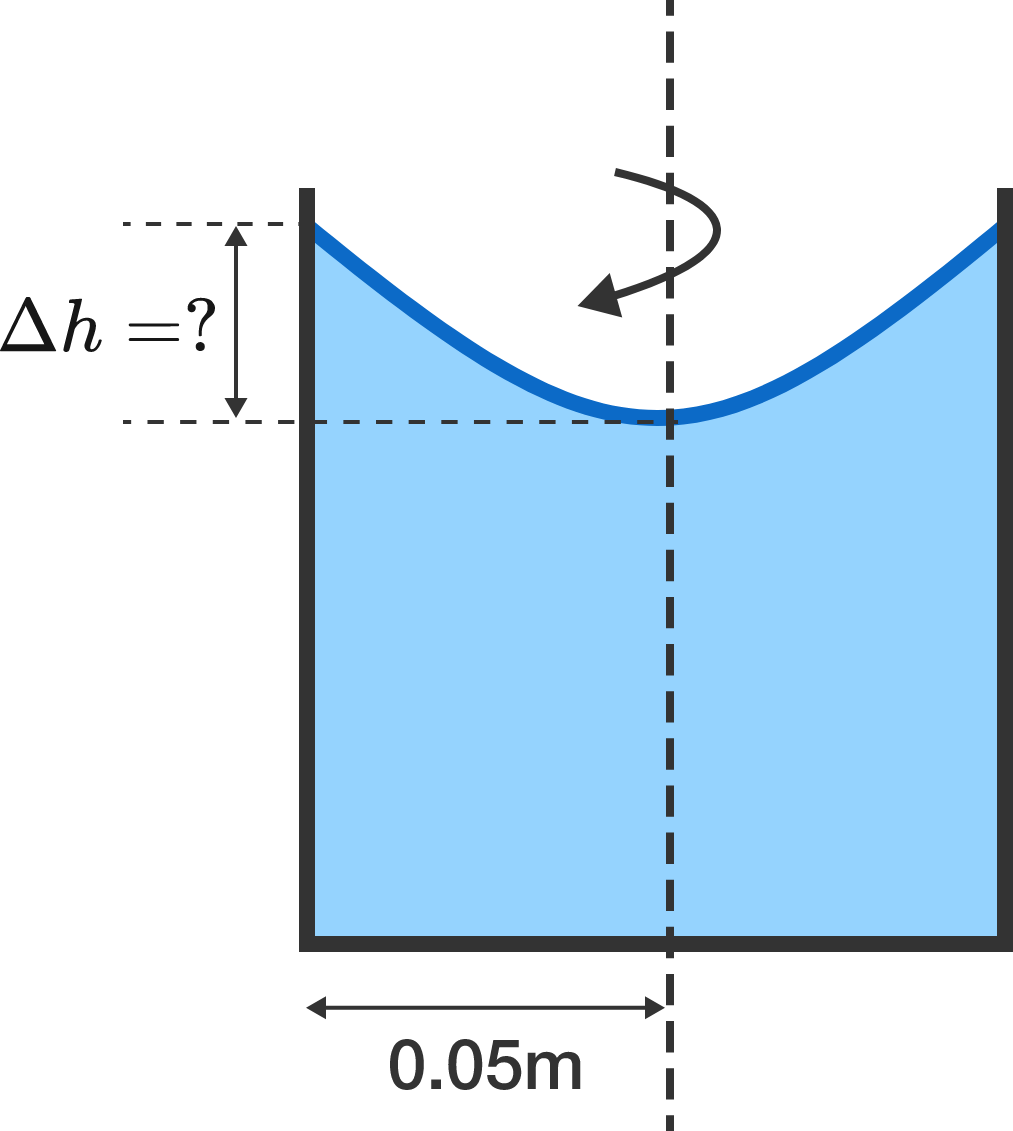# Rotate the VesselA liquid is kept in a cylindrical vessel, which is being rotated about its axis. The liquid rises at the side, as shown in the diagram.

If the radius of the vessel is $0.05\text{ m}$ and the speed of rotation is $2$ revolutions per second, find the difference in the heights of the liquid at the center of the vessel and its sides (in centimeters).

Take $g = \pi^2 \text{ m/s}^2.$

×